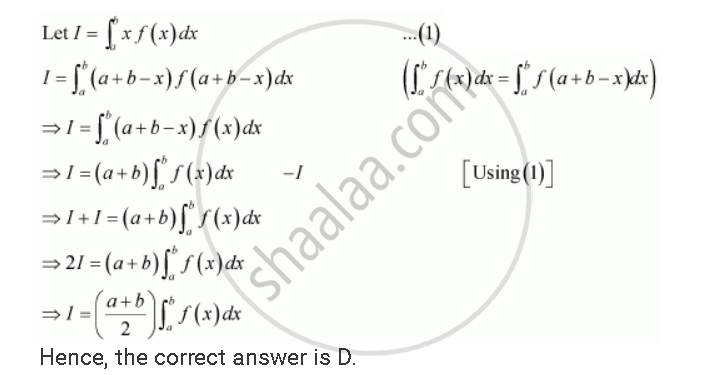Share

# Choose the Correct Answers If F (A + B – X) = F (X), Then Int_A^B X F(X )Dx is Equal - CBSE (Science) Class 12 - Mathematics

ConceptDefinite Integral as the Limit of a Sum

#### Question

Choose the correct answers If f (a + b – x) = f (x), then int_a^b x f(x )dx is equal

A (a+b)/2 int_a^b f(b-x) dx

B  (a+b)/2 int_a^b f(b+x) dx

C (b-a)/2 int_a^b f(x) dx

D (a+b)/2 int_a^b f(x) dx

#### SolutionIs there an error in this question or solution?

#### Video TutorialsVIEW ALL 

Solution Choose the Correct Answers If F (A + B – X) = F (X), Then Int_A^B X F(X )Dx is Equal Concept: Definite Integral as the Limit of a Sum.
S